# Basis (linear algebra) facts for kids

Kids Encyclopedia Facts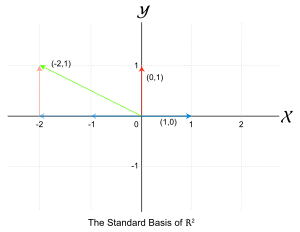This picture illustrates the standard basis in R2. The red and blue vectors are the elements of the basis; the green vector can be given with the basis vectors.

In linear algebra, a basis is a set of vectors in a given vector space with certain properties:

• One can get any vector in the vector space by multiplying each of the basis vectors by different numbers, and then adding them up.
• If any vector is removed from the basis, the property above is no longer satisfied.

The Dimension of a given vector space is the number of elements of the basis.

## Example

If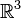$\mathbb{R}^3$ is the vector space then :

B$=${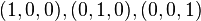$(1,0,0),(0,1,0),(0,0,1)$} is a basis of$\mathbb{R}^3$.

It's easy to see that for any element of$\mathbb{R}^3$ it can be represented as a combination of the above basis. Let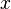$x$ be any element of$\mathbb{R}^3$, lets say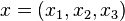$x=(x_1,x_2,x_3)$

Since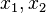$x_1,x_2$ and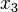$x_3$ are elements of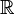$\mathbb{R}$ then they can be written as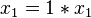$x_1=1*x_1$ and so on.

Then the combination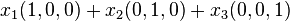$x_1(1,0,0) + x_2(0,1,0) + x_3(0,0,1)$ equals the element$x$

This shows that the set B is a basis of$\mathbb{R}^3$

## Images for kidsBasis (linear algebra) Facts for Kids. Kiddle Encyclopedia.